Ex 7.2

Chapter 7 Class 10 Coordinate Geometry
Serial order wise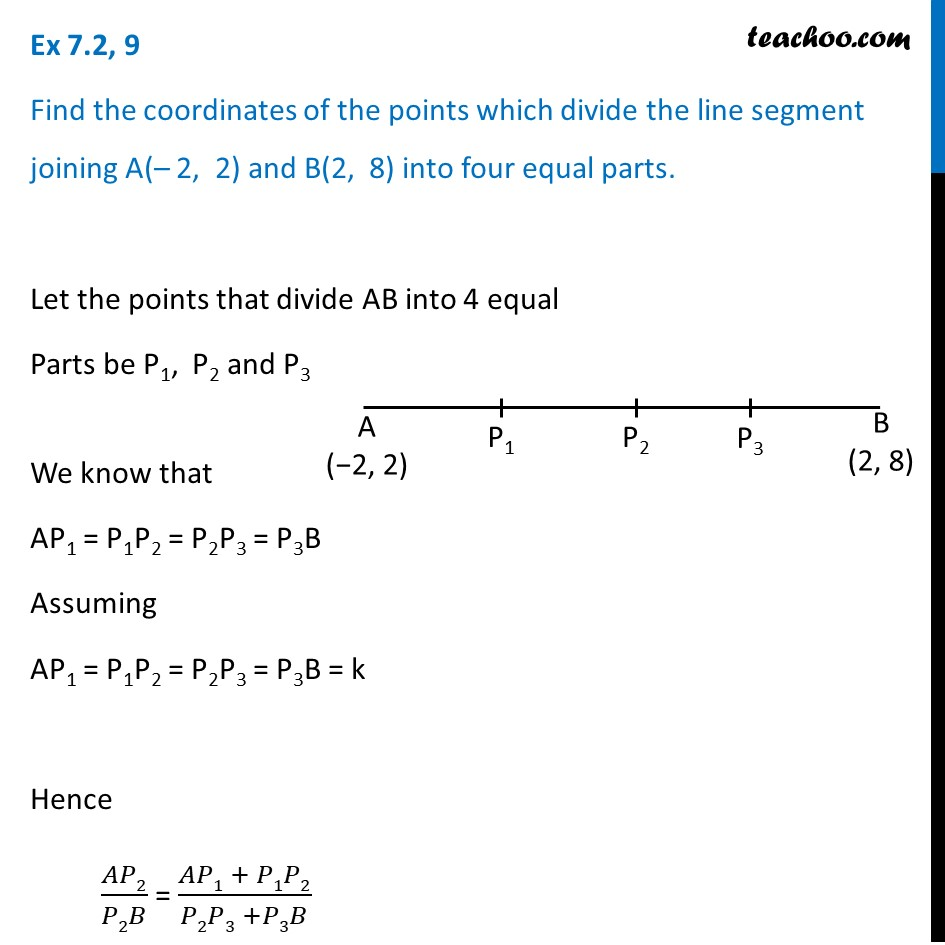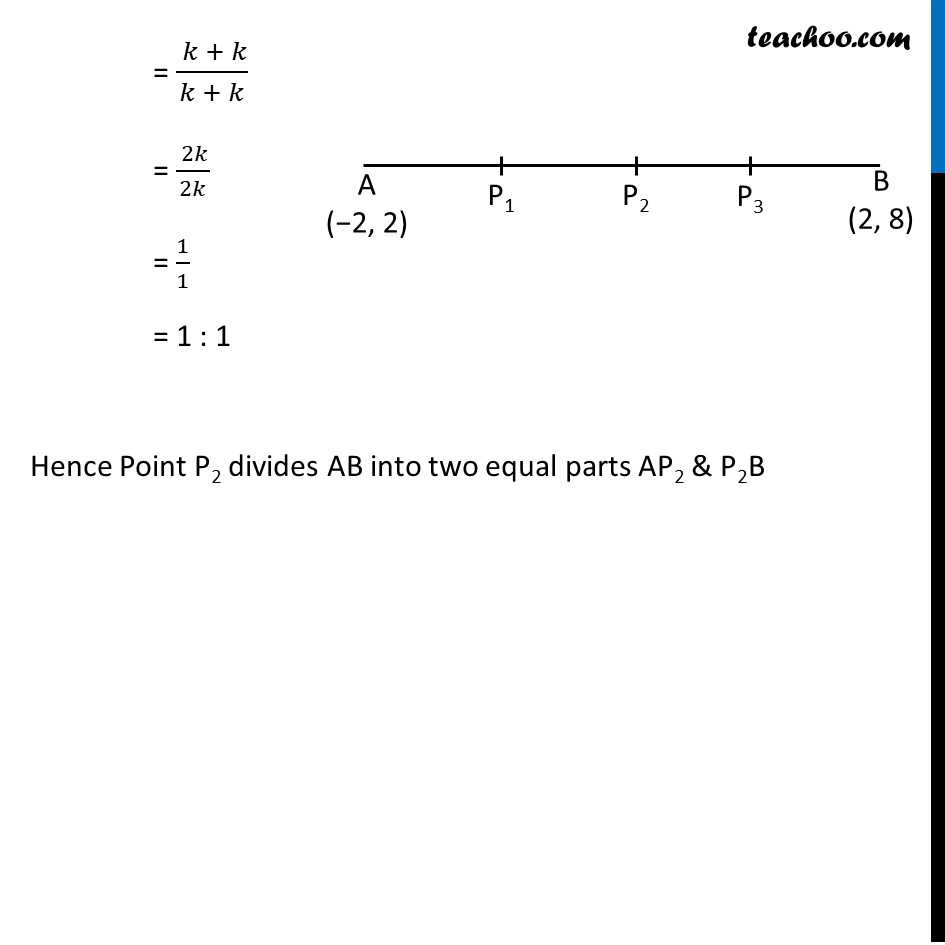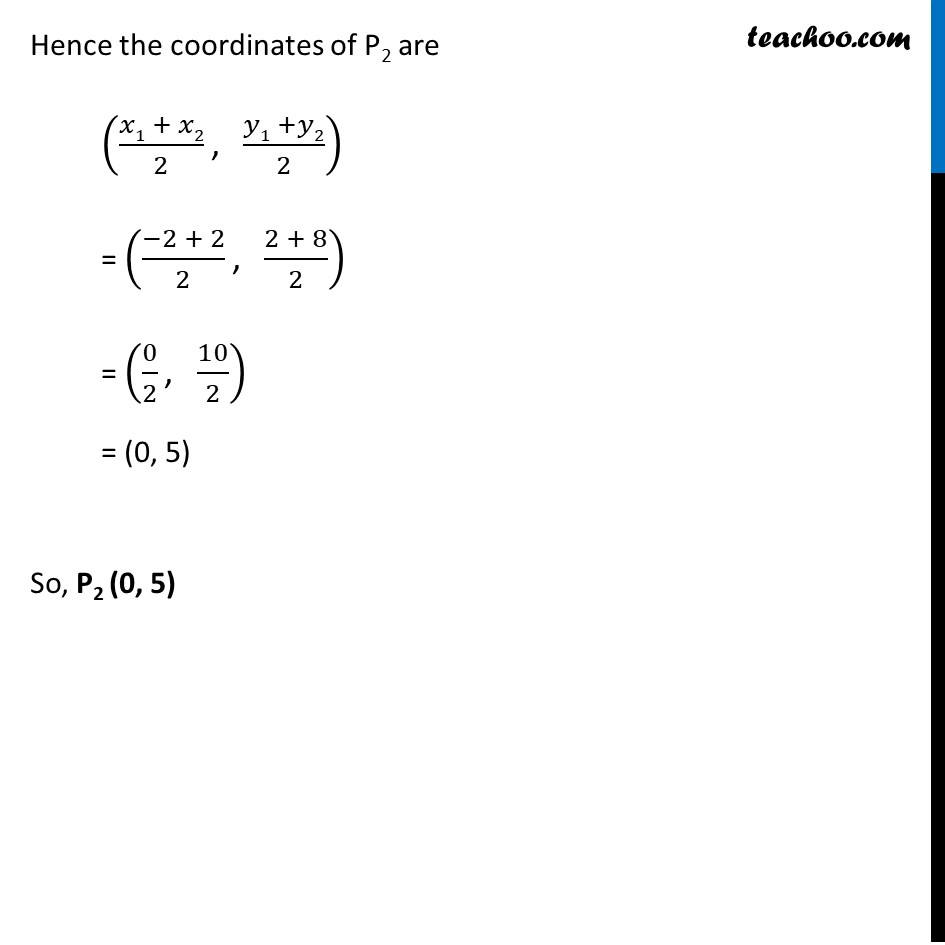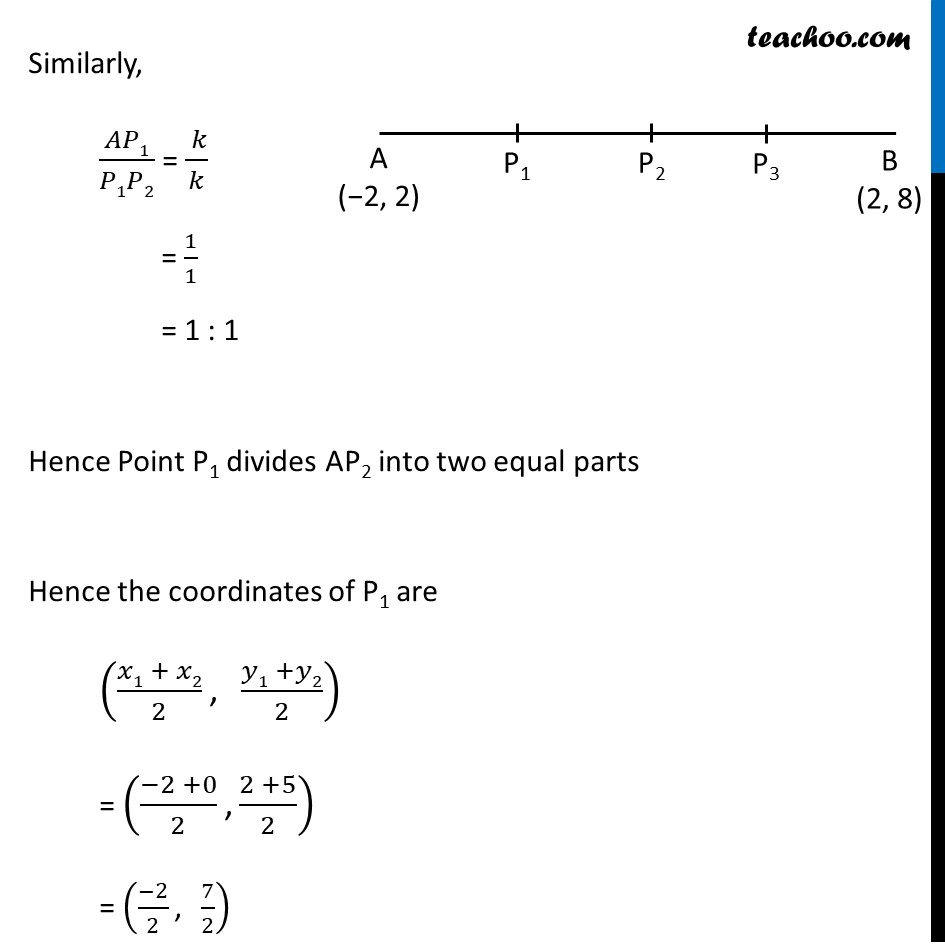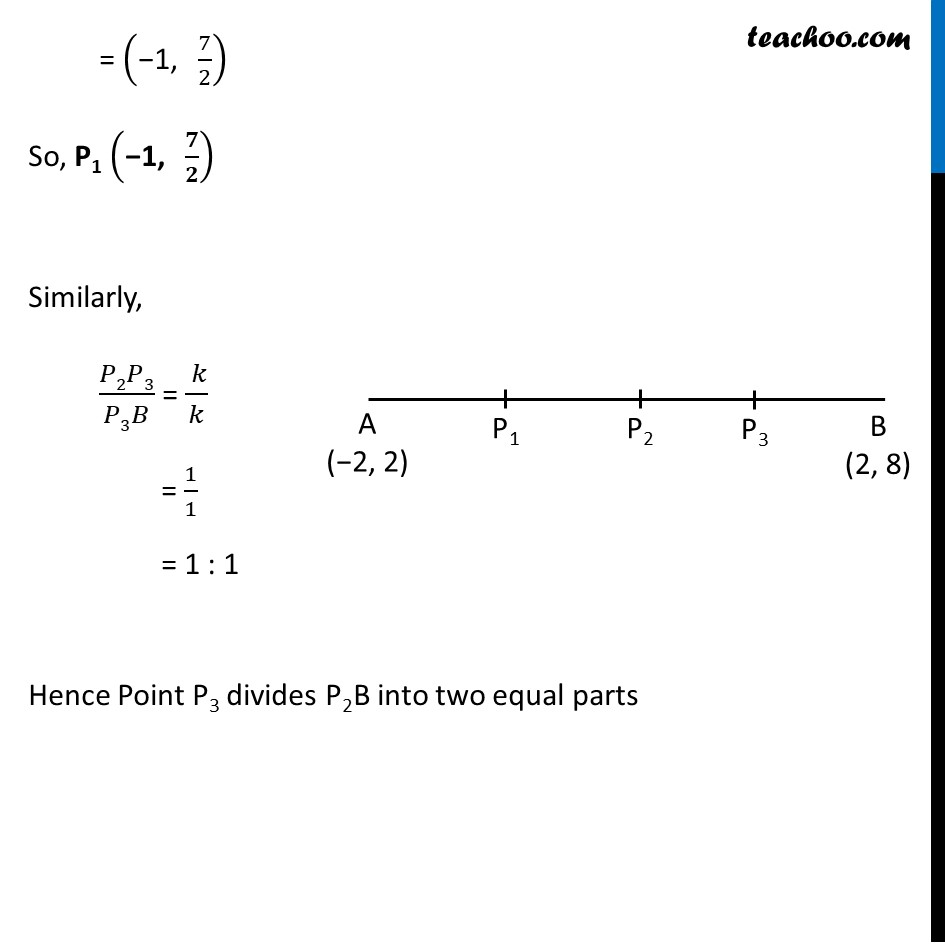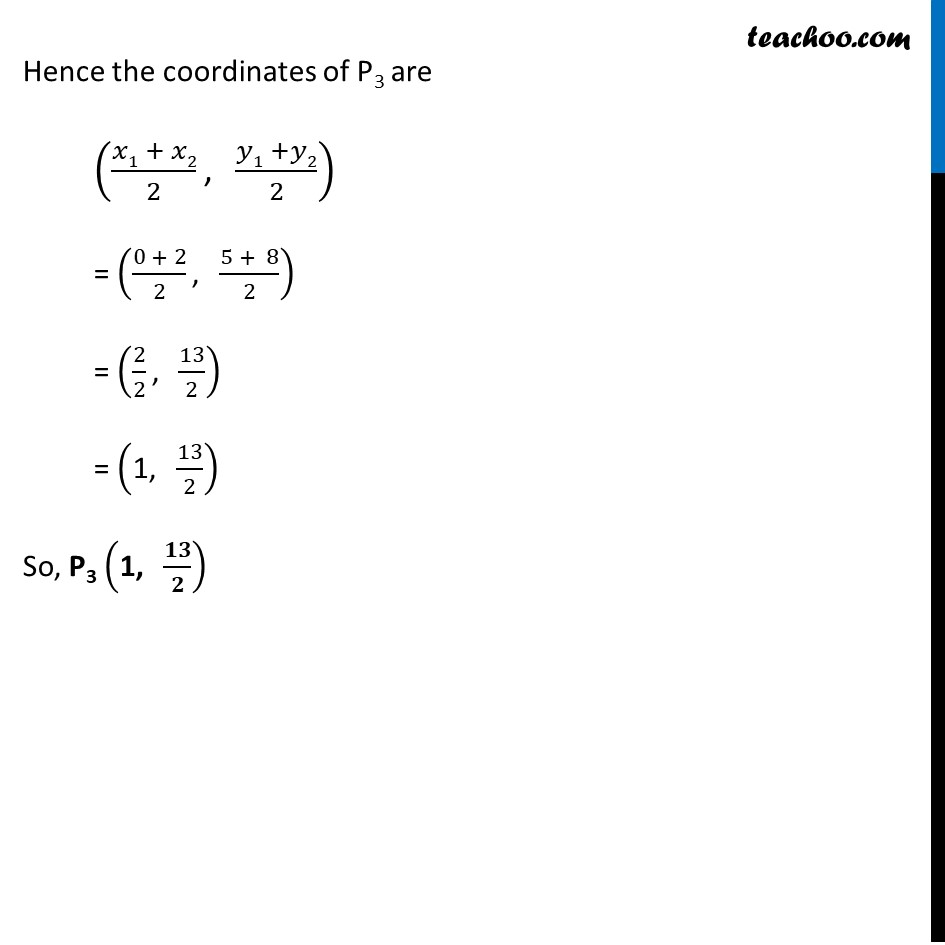Learn in your speed, with individual attention - Teachoo Maths 1-on-1 Class

### Transcript

Ex 7.2, 9 Find the coordinates of the points which divide the line segment joining A(– 2, 2) and B(2, 8) into four equal parts. Let the points that divide AB into 4 equal Parts be P1, P2 and P3 We know that AP1 = P1P2 = P2P3 = P3B Assuming AP1 = P1P2 = P2P3 = P3B = k Hence 𝐴𝑃2/𝑃2𝐵 = (𝐴𝑃1 + 𝑃1𝑃2)/(𝑃2𝑃3 +𝑃3𝐵) = ( 𝑘 + 𝑘)/(𝑘 + 𝑘) = ( 2𝑘)/2𝑘 = 1/1 = 1 : 1 Hence Point P2 divides AB into two equal parts AP2 & P2B Hence the coordinates of P2 are ((𝑥1 + 𝑥2)/2 ", " (𝑦1 +𝑦2)/2) = ((−2 + 2)/2 ", " (2 + 8)/2) = (0/2 ", " 10/2) = (0, 5) So, P2 (0, 5) Similarly, 𝐴𝑃1/𝑃1𝑃2 = ( 𝑘)/𝑘 = 1/1 = 1 : 1 Hence Point P1 divides AP2 into two equal parts Hence the coordinates of P1 are ((𝑥1 + 𝑥2)/2 ", " (𝑦1 +𝑦2)/2) = ((−2 +0)/2 "," (2 +5)/2) = ((−2)/2 ", " 7/2) = ("−1, " 7/2) So, P1 ("−1, " 𝟕/𝟐) Similarly, 𝑃2𝑃3/𝑃3𝐵 = ( 𝑘)/𝑘 = 1/1 = 1 : 1 Hence Point P3 divides P2B into two equal parts Hence the coordinates of P3 are ((𝑥1 + 𝑥2)/2 ", " (𝑦1 +𝑦2)/2) = ((0 + 2)/2 ", " (5 + 8)/2) = (2/2 ", " 13/2) = ("1, " 13/2) So, P3 ("1, " 𝟏𝟑/𝟐)